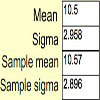#### You may also like### Spinners

How do scores on dice and factors of polynomials relate to each other?### Data Matching

Use your skill and judgement to match the sets of random data.### Random Inequalities

Can you build a distribution with the maximum theoretical spread?

# Time to Evolve 2

### Why do this problem?

This task involves investigating pdfs and modelling though the uniform distribution. It combines some theoretical analysis of mean and variance and can be used to create an intuition that the sum of random variables tends to a normal distribution with the aid of a spreadsheet simulation. It is a common misconception that the sum of uniform random variables is also uniform and this problem will allow students to see that this is not the case in a meaningful context.

### Possible approach

This problem could easily be done individually, but questions concerning the soundness of the modelling assumptions would suit themselves to group discussion.

One of the main obstacles to getting into this problem is the technical formalism. Although the symbolism is very natural, learners will need to be encouraged to read the question carefully and think how the words relate to the symbols and why this setup makes sense.

### Key questions

What is Var$(X+Y)$? What is Var$(aX)$?
Why is the sum of two uniform random variables not uniform?
Could we use a variant of this idea to model the time between the births and deaths of humans?

### Possible extension

Students, for any problem, can always:
It is often surprising to people that there is no nice analytic expression for the sum of a set of uniform distributions. The web link mentioned in the foot of the problem would lead the interested student to many nice results.

### Possible support

Students struggling to get started could try the easier, related, question time to evolve .